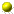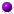# CONVERSIONS: METRIC vs U.S.

##temperature

• °F = °C x 9/5 + 32 or °C x 1.8 + 32
example 212°F = 100°C x 9/5 + 32 or 100°C x 1.8 + 32
• °C = °F -32 x 5/9 or °F -32 x .555
example 100°C = 212°F -32 x 5/9 or 212°F - 32 x .555

##distance

• miles = kms. x 5/8 or kms. x .62 (approx.)
example 5 miles = 8 kms. x 5/8 or 8 kms. x .62
• kilometers (kms) = miles x 8/5 or miles x 1.6
example 8 kms. = 5 miles x 8/5 or 8 kms. = 5 miles x 1.6

##measurement

• feet = meters x 3.3 (approx.)
example 330 ft. = 100 meters x 3.3
• meters = feet x .3 (approx.)
example 100 meters = 330 ft. x .3

##weight

• 1 ounce (oz.) = 28.35 grams (gms.)
• 1 gram (gm.) = .035 ounces (ozs.)
• 1 pound (lb.) = .45 kilograms (kgs.)
• 1 kilogram (kg.) = 2.2 pounds (lbs.)

##volume

• 1 quart (qt.) = .95 liter
• 1 liter = 1.06 quarts (qts.)
• 1 gallon (gal.) = 3.8 liters
• 1 liter = . 26 gallons (gals.)Travel Info